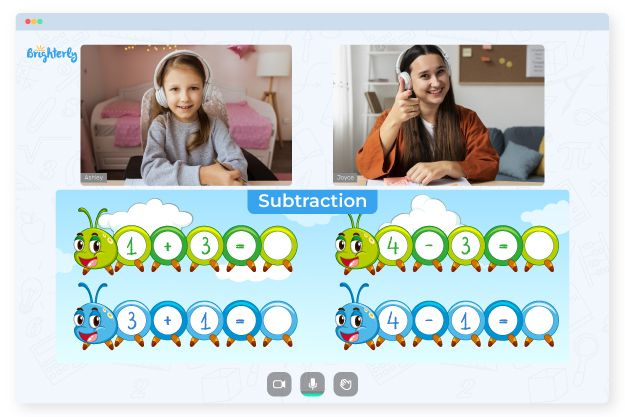# Subtracting Decimals Worksheets

The numbers with a decimal point are referred to as decimal numbers. They usually have two parts: the number of decimal places remaining and the fractional portion (right of the decimal point). Kids could have issues understanding how to subtract decimals, so a subtracting decimals worksheet becomes a useful tool in their education.

## Subtracting Decimals Worksheets from Brighterly

Brighterly is a platform that can be used to help kids understand elementary math better. For example, to explain subtraction of decimals from a number, Brighterly’s tutors use subtracting decimals worksheets. They help pupils arrange the digits in a row so that decimal points are evenly spaced apart. If required, tutors explain to kids how to use zeros as placeholders to ensure each column has numbers.

Kids have to subtract from right to left and proceed to write the decimal point below the decimal point in the subtrahend. Completing exercises in subtract decimals worksheet, kids must always align decimal points so that the place-value locations fall in place. They should regroup and perform subtraction just as they would with whole numbers.

Math for Kids

Is Your Child Struggling With Math?
1:1 Online Math TutoringReal-world decimal issues, such as those involving money, may be solved using these techniques.

## How to Use Subtracting Decimals Worksheets in a Fun Way

Typically, a decimal subtraction worksheet includes monetary calculations of dollars and cents because the ability to work with decimal numbers is vital for dealing with money. For instance, if your kids were to go to the local grocery store to purchase items worth 53.83, and the kids give the vendor 60 dollars, the balance would be 6.17.### Subtracting Decimals Worksheets PDF

Subtracting Decimals Worksheet### Subtracting Decimals Worksheets PDF

Decimal Subtraction Worksheet### Subtracting Decimals Worksheets PDF

Subtract Decimals Worksheet### Subtracting Decimals Worksheets PDF

Subtraction Of Decimals Worksheet

Kids often have to deal with decimals since dollars and cents are generally expressed in decimal forms. Before getting items from the grocery shop, kids need a thorough understanding of decimal numbers. If they were to make a mistake, they may lose a lot of money.

So, understanding place value is critical for adding or subtracting decimal numbers. Using a subtraction of decimals worksheet, kids can start from the basics with a simple example that explains this concept before going on to more complex problems.

### More Decimals Worksheets

Struggling with Subtraction?• Does your child struggle to grasp subtraction?
• Try studying with an online tutor.

Is your child having difficulties understanding subtraction lessons? An online tutor could provide the necessary guidance.